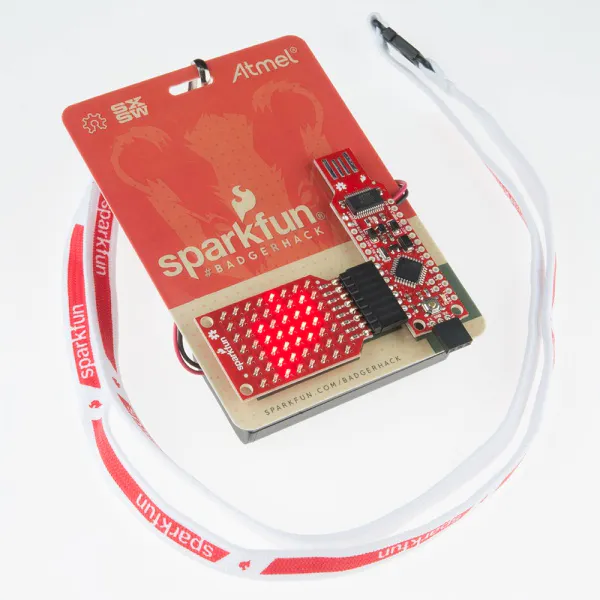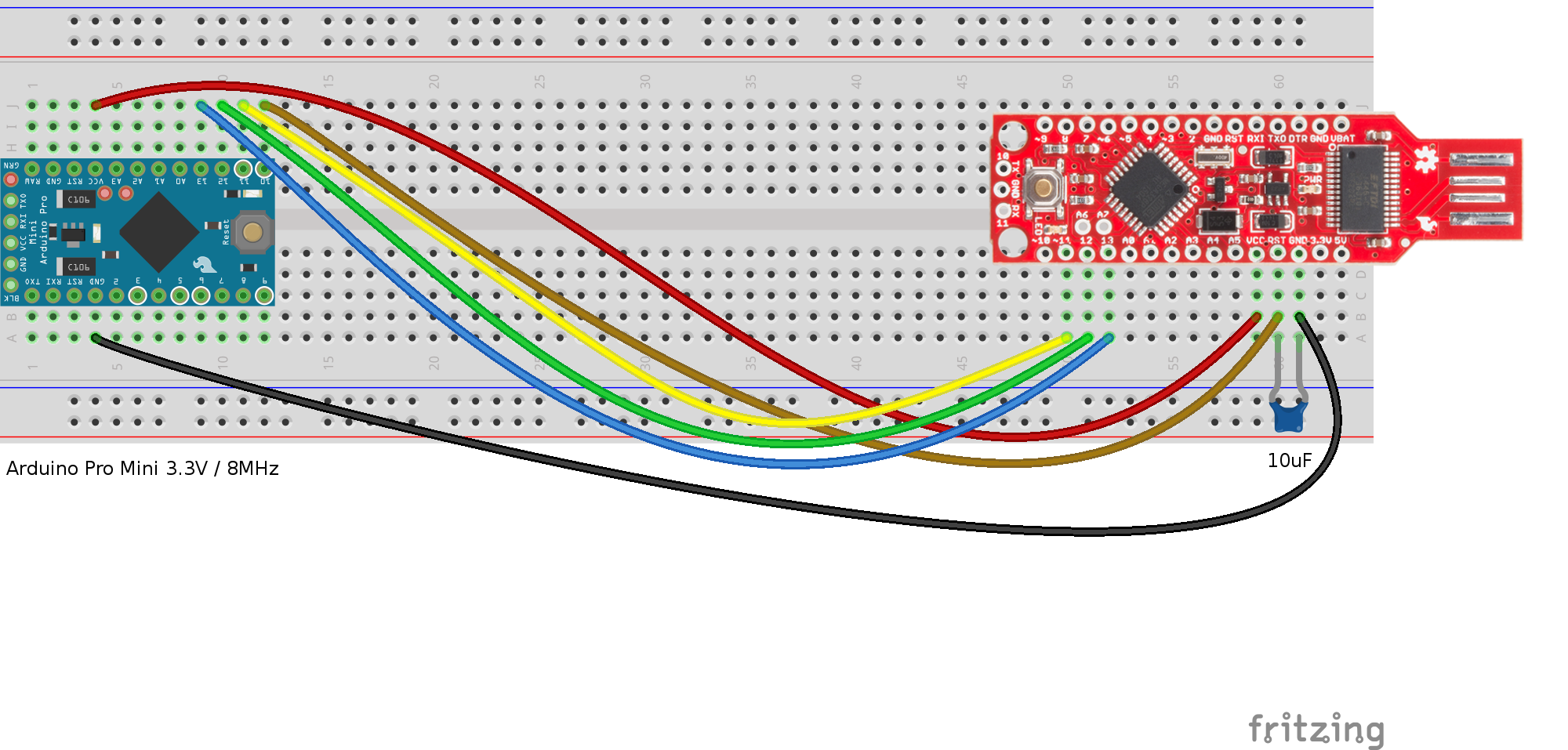Project tutorialHow to upgrade the BadgerStick Arduino board by SparkFun, if you got one at SXSW 2015

• 1,961 views
• 6 respects

## Apps and online services

 codebender

This tutorial will help you program an old BadgerStick (from SXSW 2015) with a new bootloader, so that you can then use it as a regular Arduino device and load new sketches on it.

First of all, let's explain what a bootloader is. In a nutshell, it's a small program that runs when your Arduino (or other similar device) first powers up. This is responsible for talking to your computer to see if you are trying to program a new sketch, and if so, accepts the new sketch.

#### What's the issue?

The first batch of BadgerSticks that were distributed in SXSW 2015 were programmed with an old version of the bootloader, that works at a very slow speed. This is no longer supported, so we need to re-program, or "flash" the new bootloader to our BadgerStick.

#### Fixing the issue

To flash the new bootloader to the BadgerStick, you will need something called an ISP programmer. If you already have one and know how to use it, just move on to the last section. If you don't have an ISP programmer, you can set up an Arduino to act like one. In this case, we will use an Arduino Pro Mini, but you can also use an Arduino UNO if you want.

First of all, you will need to program your Arduino with the ArduinoISP sketch, which is the code embedded below. This will make your Arduino act as an ISP programmer.

Go to the code section below, and install the codebender browser using the Learn More link on the bottom right. This plugin allows your browser to talk to your Arduino, and program it with new sketches.

If you already have the codebender browser plugin, just select your Arduino device, select the right Serial (COM) port, and click Run on Arduino. After a few seconds, you should get a success message. This means your Arduino is now set up to act as an ISP programmer.

Note: We assume that you already have the necessary FTDI drivers installed on your computer. If not, I encourage you to go to codebender's Getting Started Walkthrough which will help you set up and configure your computer.

Use the schematic below to connect your Arduino to your BadgerStick. Again, this shows an Arduino Pro Mini, but you can also use an UNO with the same pins.

Now go to codebender's Burn Bootloader page.

Select the programmer. If you already have one, select the type you have. If you have been following this tutorial, select ArduinoISP.

If you selected ArduinoISP, another selection box will appear asking you to select a Serial (COM) port. Select the one that belongs to your Arduino.

Click Burn Bootloader. After a while, a success message will appear.

Try it out, program this Hello World sketch.

## Code

##### Untitled fileArduino
```// ArduinoISP version 04m3
// Copyright (c) 2008-2011 Randall Bohn
// If you require a license, see
//
// This sketch turns the Arduino into a AVRISP
// using the following arduino pins:
//
// pin name:    not-mega:         mega(1280 and 2560)
// slave reset: 10:               53
// MOSI:        11:               51
// MISO:        12:               50
// SCK:         13:               52
//
// Put an LED (with resistor) on the following pins:
// 9: Heartbeat   - shows the programmer is running
// 8: Error       - Lights up if something goes wrong (use red if that makes sense)
// 7: Programming - In communication with the slave
//
// 23 July 2011 Randall Bohn
// -Address Arduino issue 509 :: Portability of ArduinoISP
//
// October 2010 by Randall Bohn
// - Write to EEPROM > 256 bytes
// - Better use of LEDs:
// -- Flash LED_PMODE on each flash commit
// -- Flash LED_PMODE while writing EEPROM (both give visual feedback of writing progress)
// - Light LED_ERR whenever we hit a STK_NOSYNC. Turn it off when back in sync.
// - Use pins_arduino.h (should also work on Arduino Mega)
//
// October 2009 by David A. Mellis
//
// February 2009 by Randall Bohn
// - Added support for writing to EEPROM (what took so long?)
// Windows users should consider WinAVR's avrdude instead of the
// avrdude included with Arduino software.
//
// January 2008 by Randall Bohn
// - Thanks to Amplificar for helping me with the STK500 protocol
// - The AVRISP/STK500 (mk I) protocol is used in the arduino bootloader
// - The SPI functions herein were developed for the AVR910_ARD programmer

#include "pins_arduino.h"
#define RESET     SS

#define LED_HB    9
#define LED_ERR   8
#define LED_PMODE 7
#define PROG_FLICKER true

#define HWVER 2
#define SWMAJ 1
#define SWMIN 18

// STK Definitions
#define STK_OK      0x10
#define STK_FAILED  0x11
#define STK_UNKNOWN 0x12
#define STK_INSYNC  0x14
#define STK_NOSYNC  0x15
#define CRC_EOP     0x20 //ok it is a space...

void pulse(int pin, int times);

void setup() {
Serial.begin(19200);
pinMode(LED_PMODE, OUTPUT);
pulse(LED_PMODE, 2);
pinMode(LED_ERR, OUTPUT);
pulse(LED_ERR, 2);
pinMode(LED_HB, OUTPUT);
pulse(LED_HB, 2);
}

int error=0;
int pmode=0;
int here;
uint8_t buff; // global block storage

typedef struct param {
uint8_t devicecode;
uint8_t revision;
uint8_t progtype;
uint8_t parmode;
uint8_t polling;
uint8_t selftimed;
uint8_t lockbytes;
uint8_t fusebytes;
int flashpoll;
int eeprompoll;
int pagesize;
int eepromsize;
int flashsize;
}
parameter;

parameter param;

// this provides a heartbeat on pin 9, so you can tell the software is running.
uint8_t hbval=128;
int8_t hbdelta=8;
void heartbeat() {
if (hbval > 192) hbdelta = -hbdelta;
if (hbval < 32) hbdelta = -hbdelta;
hbval += hbdelta;
analogWrite(LED_HB, hbval);
delay(20);
}

void loop(void) {
// is pmode active?
if (pmode) digitalWrite(LED_PMODE, HIGH);
else digitalWrite(LED_PMODE, LOW);
// is there an error?
if (error) digitalWrite(LED_ERR, HIGH);
else digitalWrite(LED_ERR, LOW);

// light the heartbeat LED
heartbeat();
if (Serial.available()) {
avrisp();
}
}

uint8_t getch() {
while(!Serial.available());
}
void fill(int n) {
for (int x = 0; x < n; x++) {
buff[x] = getch();
}
}

#define PTIME 30
void pulse(int pin, int times) {
do {
digitalWrite(pin, HIGH);
delay(PTIME);
digitalWrite(pin, LOW);
delay(PTIME);
}
while (times--);
}

void prog_lamp(int state) {
if (PROG_FLICKER)
digitalWrite(LED_PMODE, state);
}

void spi_init() {
uint8_t x;
SPCR = 0x53;
x=SPSR;
x=SPDR;
}

void spi_wait() {
do {
}
while (!(SPSR & (1 << SPIF)));
}

uint8_t spi_send(uint8_t b) {
SPDR=b;
spi_wait();
}

uint8_t spi_transaction(uint8_t a, uint8_t b, uint8_t c, uint8_t d) {
uint8_t n;
spi_send(a);
n=spi_send(b);
//if (n != a) error = -1;
n=spi_send(c);
return spi_send(d);
}

if (CRC_EOP == getch()) {
Serial.print((char)STK_INSYNC);
Serial.print((char)STK_OK);
}
else {
error++;
Serial.print((char)STK_NOSYNC);
}
}

if (CRC_EOP == getch()) {
Serial.print((char)STK_INSYNC);
Serial.print((char)b);
Serial.print((char)STK_OK);
}
else {
error++;
Serial.print((char)STK_NOSYNC);
}
}

void get_version(uint8_t c) {
switch(c) {
case 0x80:
break;
case 0x81:
break;
case 0x82:
break;
case 0x93:
break;
default:
}
}

void set_parameters() {
// call this after reading paramter packet into buff[]
param.devicecode = buff;
param.revision   = buff;
param.progtype   = buff;
param.parmode    = buff;
param.polling    = buff;
param.selftimed  = buff;
param.lockbytes  = buff;
param.fusebytes  = buff;
param.flashpoll  = buff;
// ignore buff (= buff)
// following are 16 bits (big endian)
param.eeprompoll = beget16(&buff);
param.pagesize   = beget16(&buff);
param.eepromsize = beget16(&buff);

// 32 bits flashsize (big endian)
param.flashsize = buff * 0x01000000
+ buff * 0x00010000
+ buff * 0x00000100
+ buff;

}

void start_pmode() {
spi_init();
// following delays may not work on all targets...
pinMode(RESET, OUTPUT);
digitalWrite(RESET, HIGH);
pinMode(SCK, OUTPUT);
digitalWrite(SCK, LOW);
delay(50);
digitalWrite(RESET, LOW);
delay(50);
pinMode(MISO, INPUT);
pinMode(MOSI, OUTPUT);
spi_transaction(0xAC, 0x53, 0x00, 0x00);
pmode = 1;
}

void end_pmode() {
pinMode(MISO, INPUT);
pinMode(MOSI, INPUT);
pinMode(SCK, INPUT);
pinMode(RESET, INPUT);
pmode = 0;
}

void universal() {
int w;
uint8_t ch;

fill(4);
ch = spi_transaction(buff, buff, buff, buff);
}

void flash(uint8_t hilo, int addr, uint8_t data) {
spi_transaction(0x40+8*hilo,
data);
}
if (PROG_FLICKER) prog_lamp(LOW);
if (PROG_FLICKER) {
delay(PTIME);
prog_lamp(HIGH);
}
}

//#define _current_page(x) (here & 0xFFFFE0)
if (param.pagesize == 32)  return here & 0xFFFFFFF0;
if (param.pagesize == 64)  return here & 0xFFFFFFE0;
if (param.pagesize == 128) return here & 0xFFFFFFC0;
if (param.pagesize == 256) return here & 0xFFFFFF80;
return here;
}

void write_flash(int length) {
fill(length);
if (CRC_EOP == getch()) {
Serial.print((char) STK_INSYNC);
Serial.print((char) write_flash_pages(length));
}
else {
error++;
Serial.print((char) STK_NOSYNC);
}
}

uint8_t write_flash_pages(int length) {
int x = 0;
int page = current_page(here);
while (x < length) {
if (page != current_page(here)) {
commit(page);
page = current_page(here);
}
flash(LOW, here, buff[x++]);
flash(HIGH, here, buff[x++]);
here++;
}

commit(page);

return STK_OK;
}

#define EECHUNK (32)
uint8_t write_eeprom(int length) {
int start = here * 2;
int remaining = length;
if (length > param.eepromsize) {
error++;
return STK_FAILED;
}
while (remaining > EECHUNK) {
write_eeprom_chunk(start, EECHUNK);
start += EECHUNK;
remaining -= EECHUNK;
}
write_eeprom_chunk(start, remaining);
return STK_OK;
}
// write (length) bytes, (start) is a byte address
uint8_t write_eeprom_chunk(int start, int length) {
// this writes byte-by-byte,
// page writing may be faster (4 bytes at a time)
fill(length);
prog_lamp(LOW);
for (int x = 0; x < length; x++) {
delay(45);
}
prog_lamp(HIGH);
return STK_OK;
}

void program_page() {
char result = (char) STK_FAILED;
int length = 256 * getch();
length += getch();
char memtype = getch();
// flash memory @here, (length) bytes
if (memtype == 'F') {
write_flash(length);
return;
}
if (memtype == 'E') {
result = (char)write_eeprom(length);
if (CRC_EOP == getch()) {
Serial.print((char) STK_INSYNC);
Serial.print(result);
}
else {
error++;
Serial.print((char) STK_NOSYNC);
}
return;
}
Serial.print((char)STK_FAILED);
return;
}

return spi_transaction(0x20 + hilo * 8,
0);
}

for (int x = 0; x < length; x+=2) {
Serial.print((char) low);
Serial.print((char) high);
here++;
}
return STK_OK;
}

// here again we have a word address
int start = here * 2;
for (int x = 0; x < length; x++) {
int addr = start + x;
uint8_t ee = spi_transaction(0xA0, (addr >> 8) & 0xFF, addr & 0xFF, 0xFF);
Serial.print((char) ee);
}
return STK_OK;
}

char result = (char)STK_FAILED;
int length = 256 * getch();
length += getch();
char memtype = getch();
if (CRC_EOP != getch()) {
error++;
Serial.print((char) STK_NOSYNC);
return;
}
Serial.print((char) STK_INSYNC);
if (memtype == 'F') result = flash_read_page(length);
if (memtype == 'E') result = eeprom_read_page(length);
Serial.print(result);
return;
}

if (CRC_EOP != getch()) {
error++;
Serial.print((char) STK_NOSYNC);
return;
}
Serial.print((char) STK_INSYNC);
uint8_t high = spi_transaction(0x30, 0x00, 0x00, 0x00);
Serial.print((char) high);
uint8_t middle = spi_transaction(0x30, 0x00, 0x01, 0x00);
Serial.print((char) middle);
uint8_t low = spi_transaction(0x30, 0x00, 0x02, 0x00);
Serial.print((char) low);
Serial.print((char) STK_OK);
}
//////////////////////////////////////////
//////////////////////////////////////////

////////////////////////////////////
////////////////////////////////////
int avrisp() {
uint8_t data, low, high;
uint8_t ch = getch();
switch (ch) {
case '0': // signon
error = 0;
break;
case '1':
if (getch() == CRC_EOP) {
Serial.print((char) STK_INSYNC);
Serial.print("AVR ISP");
Serial.print((char) STK_OK);
}
break;
case 'A':
get_version(getch());
break;
case 'B':
fill(20);
set_parameters();
break;
case 'E': // extended parameters - ignore for now
fill(5);
break;

case 'P':
start_pmode();
break;
case 'U': // set address (word)
here = getch();
here += 256 * getch();
break;

case 0x60: //STK_PROG_FLASH
low = getch();
high = getch();
break;
case 0x61: //STK_PROG_DATA
data = getch();
break;

case 0x64: //STK_PROG_PAGE
program_page();
break;

break;

case 'V': //0x56
universal();
break;
case 'Q': //0x51
error=0;
end_pmode();
break;

break;

// expecting a command, not CRC_EOP
// this is how we can get back in sync
case CRC_EOP:
error++;
Serial.print((char) STK_NOSYNC);
break;

// anything else we will return STK_UNKNOWN
default:
error++;
if (CRC_EOP == getch())
Serial.print((char)STK_UNKNOWN);
else
Serial.print((char)STK_NOSYNC);
}
}
```

## Schematics#### Similar projects you might like

Project tutorial by Shahariar

• 1,794 views
• 8 respects

#### Arduino101 / tinyTILE BLE: Match-Making Sunglasses

Project tutorial by Kitty Yeung

• 17,264 views
• 50 respects

#### Weather Wand

Project in progress by Dan Fein

• 17,634 views
• 32 respects

#### Bluetooth Control Hat

Project showcase by EyalSch

• 15,828 views
• 60 respects

Project tutorial by Team FabLabAQ

• 6,057 views